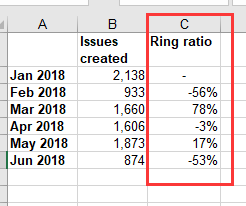# About the ring ratio calculation of data

HI，hello！
I want to calculate the ring ratio of data，Please tell me method，thank you！
ring ratio=(current moth data-last month data)/last month dataHi,

For current Month:

``````CASE
WHEN
([Time].[Month].CurrentDateMember.PrevMember,[Measures].[Issues created]) <> 0
THEN
([Measures].[Issues created] -
([Time].[Month].CurrentDateMember.PrevMember,[Measures].[Issues created])) /
([Time].[Month].CurrentDateMember.PrevMember,[Measures].[Issues created])
END
``````

and for month of your selection:

``````CASE
WHEN
([Time].CurrentHierarchyMember.PrevMember,[Measures].[Issues created]) <> 0
THEN
([Measures].[Issues created] -
([Time].CurrentHierarchyMember.PrevMember,[Measures].[Issues created])) /
([Time].CurrentHierarchyMember.PrevMember,[Measures].[Issues created])
END
``````

Gvido Neilands,
gvido@flex.bi,
eazyBI service partner

2 Likes

Hi guys,
nice topic to me, as I am trying to do something that starts from this calculation.

What about if I want to show the Ring Ratio BUT only for the current month?

In other word, I would like the system to recognize which date are we now, extrapolate the current month and then return the Ring Ratio for that specific month (only).

Is there a way to do that?

Thank you.
Andrea